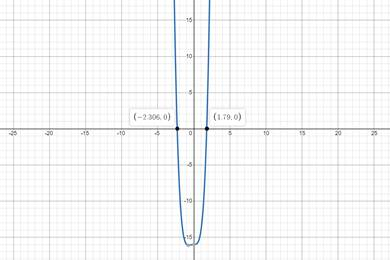# All the real solutions of given equation.### Precalculus: Mathematics for Calcu...

6th Edition
Stewart + 5 others
Publisher: Cengage Learning
ISBN: 9780840068071### Precalculus: Mathematics for Calcu...

6th Edition
Stewart + 5 others
Publisher: Cengage Learning
ISBN: 9780840068071

#### Solutions

Chapter 1.9, Problem 58E
To determine

## All the real solutions of given equation.

Expert Solution

x=2.30,1.79

### Explanation of Solution

Given information:

x4=16x3

Formula used:

Solving by making graph.

Graphically:

Making Graph of x4=16x3 and finding approximate value of x.Graph intersect x-axis at (2.30,0),(1.79,0)

x=2.30,1.79

### Have a homework question?

Subscribe to bartleby learn! Ask subject matter experts 30 homework questions each month. Plus, you’ll have access to millions of step-by-step textbook answers!